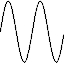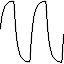# Gabotronics

The Xprotolab can output the standard waveforms of a function generator: sine, triangle and square. It can also output a custom waveform, which is initially set with an exponential.

You can adjust all the parameters of the waveform: frequency, amplitude, offset and duty cycle.

### The predefined waveforms of the AWG are:SineWave SquareWave TriangleWave CustomWave*

* The custom wave is preset with an exponential, but can be changed with the PC Xprotolab Interface.

### Frequency Sweep

The Xprotolab has a SWEEP feature, which increases the frequency of the wave automatically on each screen refresh of the oscilloscope.

The start and end frequencies are automatically determined by the current sampling rate. Since the sweep is synchronized with the oscilloscope, displaying perfect frequency plots is a breeze.When adjusting the parameters, the K1 button serves as a shortcut key, which sets predefined values.

### Technical Details

The waveform is stored in a 256 byte long buffer, this buffer is fed to the XMEGA's DAC thru the DMA. Once the waveform is set, the waveform will be generated without any CPU intervention. The maximum conversion rate of the DAC is 1MSPS, this limits the maximum output frequency of the AWG as a system. For example, if the AWG is generating a sinewave with 256 points, the maximum frequency is 3906.25Hz. If generating a sinewave with only 32 points, the maximum frequency is 31.25KHz. The AWG amplifier has a low pass filter of 44KHz.

The resolution of the generator varies depending on the frequency range: the lower the frequency, the higher the resolution:

Frequency = Cycles*125000/(Period+1)

Cycles: Integer number, with these possible values: 1,2,4,8
Period = Integer number, with values between 0 and 65535

Note that the possible frequencies are discrete.

### Testimonials

Hallo from Germany, thats a very cool item! (MultiKitB)
Michael Uhlmann